Analysis WebNotes
arrow_back arrow_forward

# Class Contents

## The Complex Numbers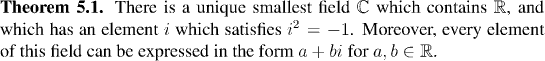Factoid:

The following properties of complex arithmetic are all deduced from the fact that C is a field, that i squares to -1 and that every element of C can be written a+bi for a,b real numbers.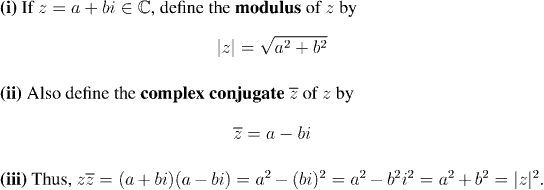## Different Types of Convergence

In previous chapters we've studied convergence in the real line quite carefully. Now we're going to look at convergence of sequences, where the sequences in question are sequences of elements of various different sets. We haven't yet had a formal definition of convergence for anything but sequences of real numbers, but the analogy with the real case will be pretty strong in these examples. We'll come to a formal definition of convergence which is broad enough to encompass all of these examples, in the next section.

Example:Formally, this definition is exactly the same as convergence in the real numbers. The difference is that the vertical lines denote the modulus of complex numbers, rather than the absolute value of real numbers. Still, the essential features are the same.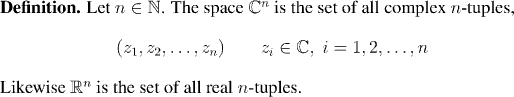Example: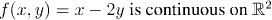On the face of it this is a pretty uncontroversial statement, but we need to have a precise statement of its means, which we now provide.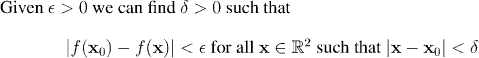Once again, this looks formally very much like the definition of continuity for real valued functions on the real numbers. However, it has to be stressed that the vertical bars now denote the Euclinean 2-norm, and so represent a more complicated quantity than simply the absolute value of a single real number. This issue will reappear when we come to actually proving that certain functions on real or complex Euclidean space are continuous.

So far we've seen examples of convergence or continuity involving real numbers, complex numbers and vectors (elements of the Euclidean spaces are often called vectors). Here's a different type of example involving convergence of a sequence whose individual terms are themselves functions.

Example: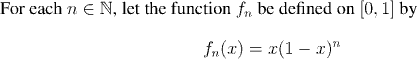Follow the link below to see an interactive demonstration of the behavior of this sequence of functions. What you'll see is that for any positive value of epsilon, however small, that you might take, eventually the graph of f_n will lie entirely below the horizontal line y=epsilon (and stay below for all subsequent values of n). We take this behavior as saying that the sequence of functions converges to zero. (In fact there are many ways that a sequence of functions might be said to converge to zero, of which this is just one. So for definiteness, we say that f_n converges to zero uniformly.)

### Click here for an interactive demo of uniform convergence

After the next definition we can make the definition of uniform convergence more congruent with our definition of convergence for real sequences.Now we can use this to give a formal definition of uniform convergence: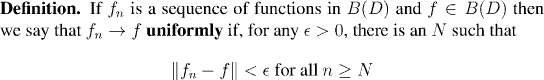This definition allows us to give some interpretation to the pictures from the interactive demonstration. When we took an epsilon line and tries to get the graph "as a whole" to lie underneath it, what we were really doing was ensuring that ||f(x)|| was smaller than epsilon

Remark:

In fact, the functions under consideration do not have to be bounded for us to talk about uniform convergence. We only insisted on it here in order to make sure that the supremums were all finite. When we come to study uniform convergence in more detail in later chapters, we will introduce another definition, which takes this into account.

The next big step is to introduce a general mathematical setup for studying convergence and continuity which is broad enough to encompass the fairly diverse types of examples we have just been looking at, together with many more that will arise. This is what we do in the next section.